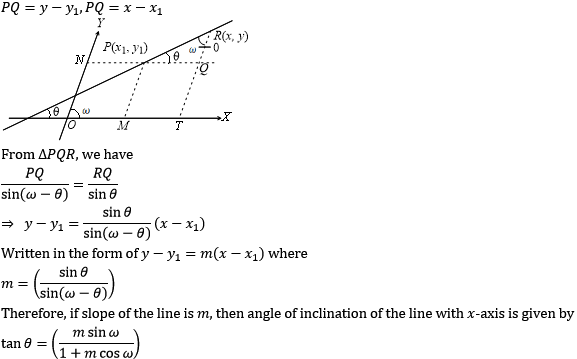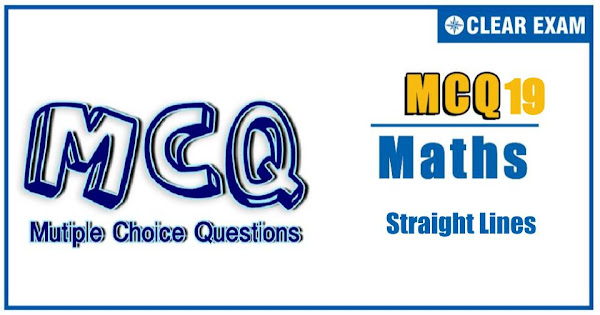## [LATEST]\$type=sticky\$show=home\$rm=0\$va=0\$count=4\$va=0

The unit Straight Line holds sheer significance in the JEE Advanced and other engineering exams. It has a weightage of 6% pairing with Circles. With focused practice good marks can be fetched from this section. These questions are important in achieving your success in JEE and Other Engineering Exams..

Q1. The locus of a moving point is the path traced out by that point under one or more given conditions. Technically, a locus represents the ‘set of points’ which lies on it.
A relation f(x,y)=0 between x and y which is satisfied by each point on the locus and such that each point satisfying the equation is on the locus is called the equation of the locus.
On the basis of above information, answer the following questions :
The locus of the point of intersection of the lines x sin⁡θ+(1-cos⁡θ )y=a sin⁡θ and x sin⁡θ-(1+cos⁡〖θ)〗 y+a sin⁡θ=0 is
•  x2-y2=a2
•  x2+y2=a2
•  y2=ax
•  None of these
Q2. Let L be the line belonging to the family of the straight lines (a+2b)x+(a-3b)y+a-8b=0,a,b∈R, which is farthest from the point (2, 2)
The equation of line L is
•  x+4y+7=0
•  2x+3y+4=0
•  4x-y-6=0
•  None of these
Q3. The equation of an altitude of an equilateral triangle is √3 x+y=2√3 and one of the vertices is (3,√3)
The possible number of triangle is
•  1
•  2
•  3
•  4

Q4. For points P=(x_1,y_1) and Q=(x_2,y_2) of the coordinate plane, a new distance d(P,Q) is defined by d(P,Q)=|x_1-x_2 |+|y_1-y_2 |. Let O=(0,0) and A=(3,2). Consider the set of points P in the first quadrant which are equidistant (with respect to the new distance) from O and A
The set of points P consists of
•  One straight line only
•  Union of two line segments
•  Union of two infinite rays
•  Union of a line segment of finite length and an infinite ray
Q5. A variable line 'L' is drawn through O(0,0) to meet the lines L1 and L2 given by y-x-10=0 and y-x-20=0 at the points A and B, respectively
A point P is taken on 'L' such that 2/OP=1/OA+1/OB. Then the locus of 'P' is
•  3x+3y=40
•  3x+3y+40=0
•  t>3x-3y=40
•  3y-3x=40
Q6. The line 6x+8y=48 intersects the coordinates axes at A and B respectively. A line L bisects the area and the perimeter of the triangle OAB where O is the origin
The number of such lines possible is
•  1
•  2
•  3
•  More than 3
Q7. A(1,3) and C(-2/5,-2/5) are the vertices of a triangle ABC and the equation of the internal angle bisector of ∠ABC is x+y=2
Equation of side BC is
•  7x+3y-4=0
•  7x+3y+4=0
•  7x-3y+4=0
•  7x-3y-4=0
Q8. Let ABCD be a parallelogram whose equations for the diagonals AC and BD are x+2y=3 and 2x+y=3, respectively. If length of diagonal AC=4 units and area of parallelogram ABCD=8 sq. units, then
The length of other diagonal BD is
•  10/3
•  2
•  20/3
•  None of these
Q9. Consider a triangle PQR with coordinates of its vertices as P(-8,5),Q(-15,-19) andR(1,-7). The bisector of the interior angle of P has the equation which can be written in the form ax+2y+c=0
The distance between the orthocentre and the circumcentre of the triangle PQR is
•  25/2
•  29/2
•  37/2
•  51/2
Q10. Let us consider the situation when axes are inclined at an angle 'ω'. If coordinates of a point P are (x1,y1), then PN=x,PM=y1, where PM is parallel to y-axis and PN is parallel x-axis. straight line through P that makes an angle θ with x-axis isThe axes being inclined at an angle of 60°, the inclination of the straight line y=2x+5 with x-axis is
•  30°
•  tan-1⁡〖(√3/2)〗
•  tan-1⁡2
•  60°#### Written by: AUTHORNAME

AUTHORDESCRIPTION## Want to know more

Please fill in the details below:

## Latest NEET Articles\$type=three\$c=3\$author=hide\$comment=hide\$rm=hide\$date=hide\$snippet=hide

Name

ltr
item
BEST NEET COACHING CENTER | BEST IIT JEE COACHING INSTITUTE | BEST NEET & IIT JEE COACHING: Straight Lines-Quiz-19
Straight Lines-Quiz-19
https://1.bp.blogspot.com/-zkMjMkkRVzc/YOQNwov6y7I/AAAAAAAAHgI/LLyi9knyuB8ylDvVshR9AbdbAoX_JtbkgCLcBGAsYHQ/s600/Quiz%2BImage%2B19.jpg
https://1.bp.blogspot.com/-zkMjMkkRVzc/YOQNwov6y7I/AAAAAAAAHgI/LLyi9knyuB8ylDvVshR9AbdbAoX_JtbkgCLcBGAsYHQ/s72-c/Quiz%2BImage%2B19.jpg
BEST NEET COACHING CENTER | BEST IIT JEE COACHING INSTITUTE | BEST NEET & IIT JEE COACHING
https://www.cleariitmedical.com/2021/07/straight-lines-quiz-19.html
https://www.cleariitmedical.com/
https://www.cleariitmedical.com/
https://www.cleariitmedical.com/2021/07/straight-lines-quiz-19.html
true
7783647550433378923
UTF-8

STAY CONNECTED